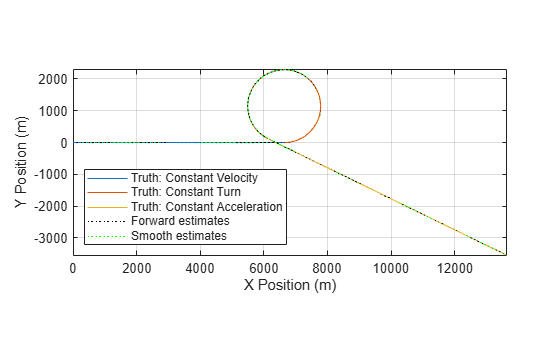# smooth

Backward smooth state estimates of `trackingIMM` filter

Since R2021a

## Syntax

``[smoothX,smoothP,modelProbability] = smooth(imm)``
``[smoothX,smoothP,modelProbability] = smooth(imm,numBackSteps)``

## Description

example

````[smoothX,smoothP,modelProbability] = smooth(imm)` runs a backward recursion to obtain smoothed states, covariances, and model probabilities at the previous steps for a `trackingIMM` filter, `imm`. The function determines the number of backward steps based on the number of executed forward steps F and the maximum number of backward steps MB specified by the `MaxNumSmoothingSteps` property of the `filter`. If F < MB, the number of backward steps is F – 1. Otherwise, the number of backward steps is MB. The number of forward steps is equal to the number of calls to the `predict` object function of the filter. The backward steps do not include the current time step of the filter.```
````[smoothX,smoothP,modelProbability] = smooth(imm,numBackSteps)` specifies the number of backward smoothing steps `numBackSteps`. The value of `numBackSteps` must be less than or equal to the smaller of F – 1 and MB, where F is the number of executed forward steps and MB is the maximum number of backward steps specified by the `MaxNumSmoothingSteps` property of the filter. ```

## Examples

collapse all

Generate a truth using the attached `helperGenerateTruth` function. The truth trajectory consists of constant velocity, constant acceleration, and constant turn trajectory segments.

```n = 1000; [trueState, time, fig1] = helperGenerateTruth(n); dt = diff(time(1:2)); numSteps = numel(time);```

Set up the initial conditions for the simulation.

```rng(2021); % for repeatable results positionSelector = [1 0 0 0 0 0; 0 0 1 0 0 0; 0 0 0 0 1 0]; % Select position from 6-dimenstional state [x;vx;y;vy;z;vz] truePos = positionSelector*trueState; sigma = 10; % Measurement noise standard deviation measNoise = sigma* randn(size(truePos)); measPos = truePos + measNoise; initialState = positionSelector'*measPos(:,1); initialCovariance = diag([1 1e4 1 1e4 1 1e4]); % Velocity is not measured```

Construct an IMM filter based on three EKF filters and initialize the IMM filter.

```detection = objectDetection(0,[0; 0; 0],'MeasurementNoise',sigma^2 * eye(3)); f1 = initcvekf(detection); % constant velocity EKF f2 = initcaekf(detection); % constant acceleration EKF f2.ProcessNoise = 3*eye(3); f3 = initctekf(detection); % Constant turn EKF f3.ProcessNoise(3,3) = 100; imm = trackingIMM({f1; f2; f3},'TransitionProbabilities',0.99, ... 'ModelConversionFcn',@switchimm, ... 'EnableSmoothing',true, ... 'MaxNumSmoothingSteps',size(measPos,2)-1); initialize(imm, initialState, initialCovariance);```

Preallocate variables for filter outputs.

```estState = zeros(6,numSteps); modelProbs = zeros(numel(imm.TrackingFilters),numSteps); modelProbs(:,1) = imm.ModelProbabilities;```

Run the filter.

```for i = 2:size(measPos,2) predict(imm,dt); estState(:,i) = correct(imm,measPos(:,i)); modelProbs(:,i) = imm.ModelProbabilities; end```

Smooth the filter.

`[smoothState,~,smoothProbs] = smooth(imm);`

Visualize the estimates. Zoom in on the figure to view details.

`fig1`
```fig1 = Figure (1) with properties: Number: 1 Name: '' Color: [1 1 1] Position: [348 376 583 437] Units: 'pixels' Show all properties ```
```hold on; plot3(estState(1,:),estState(3,:),estState(5,:),'k:','DisplayName','Forward estimates') plot3(smoothState(1,:),smoothState(3,:),smoothState(5,:),'g:','DisplayName','Smooth estimates') axis image;```Plot the estimate errors for both the forward estimated states and the smoothed states. From the results, the smoothing process reduces the estimation errors.

```figure; errorTruth = abs(estState - trueState); errorSmooth = abs(smoothState - trueState(:,1:end-1)); subplot(3,1,1) plot(time,errorTruth(1,:),'k') hold on plot(time(1:end-1),errorSmooth(1,:),'g') ylabel('x-error (m)') legend('Forward estimate error','Smooth error') title('Absolute Error of Forward and Smooth Estimates') subplot(3,1,2) plot(time,errorTruth(2,:),'k') hold on; plot(time(1:end-1),errorSmooth(2,:),'g') ylim([0 30]) ylabel('y-error (m)') subplot(3,1,3) plot(time,errorTruth(3,:),'k') hold on plot(time(1:end-1),errorSmooth(3,:),'g') xlabel('time (sec)') ylabel('z-error (m)')```Show the model probabilities based on forward filtering and backward smoothing.

```figure plot(time,modelProbs(1,:)) hold on plot(time,modelProbs(2,:)) plot(time,modelProbs(3,:)) xlabel('Time (sec)') title('Model Probabilities in Forward Filtering') legend('Constant velocity','Constant turn','Constant acceleration')``````figure plot(time(1:end-1),smoothProbs(1,:)) hold on plot(time(1:end-1),smoothProbs(2,:)) plot(time(1:end-1),smoothProbs(3,:)) xlabel('Time (sec)'); title('Model Probabilities in Backward Smoothing') legend('Constant velocity','Constant turn','Constant acceleration')```## Input Arguments

collapse all

Filter for object tracking, specified as an `trackingIMM` object.

Number of backward steps, specified as a positive integer. The value must be less than or equal to the smaller of F – 1 and MB, where F is the number of executed forward steps and MB is the maximum number of backward steps specified by the `MaxNumSmoothingSteps` property of the filter, `imm`.

## Output Arguments

collapse all

Smoothed states, returned as an N-by-K matrix. N is the state dimension and K is the number of backward steps. The first column represents the state at the end of backward recursion, which is the earliest state in the time interval of smoothing. The last column represents the state at the beginning of backward recursion, which is the latest state in the time interval of smoothing.

Data Types: `single` | `double`

Smoothed covariances, returned as an N-by-N-by-K array. N is the state dimension and K is the number of backward steps. Each page (an N-by-N matrix) of the array is the smoothed covariance matrix for the corresponding smoothed state in the `smoothX` output.

Data Types: `single` | `double`

Probability of each model, returned as a K-by-M array of nonnegative scalars. K is the number of backward steps and M is the number of models used in the filter.

Data Types: `single` | `double`

 Nadarajah, N., R. Tharmarasa, Mike McDonald, and T. Kirubarajan. “IMM Forward Filtering and Backward Smoothing for Maneuvering Target Tracking.” IEEE Transactions on Aerospace and Electronic Systems 48, no. 3 (July 2012): 2673–78. https://doi.org/10.1109/TAES.2012.6237617.